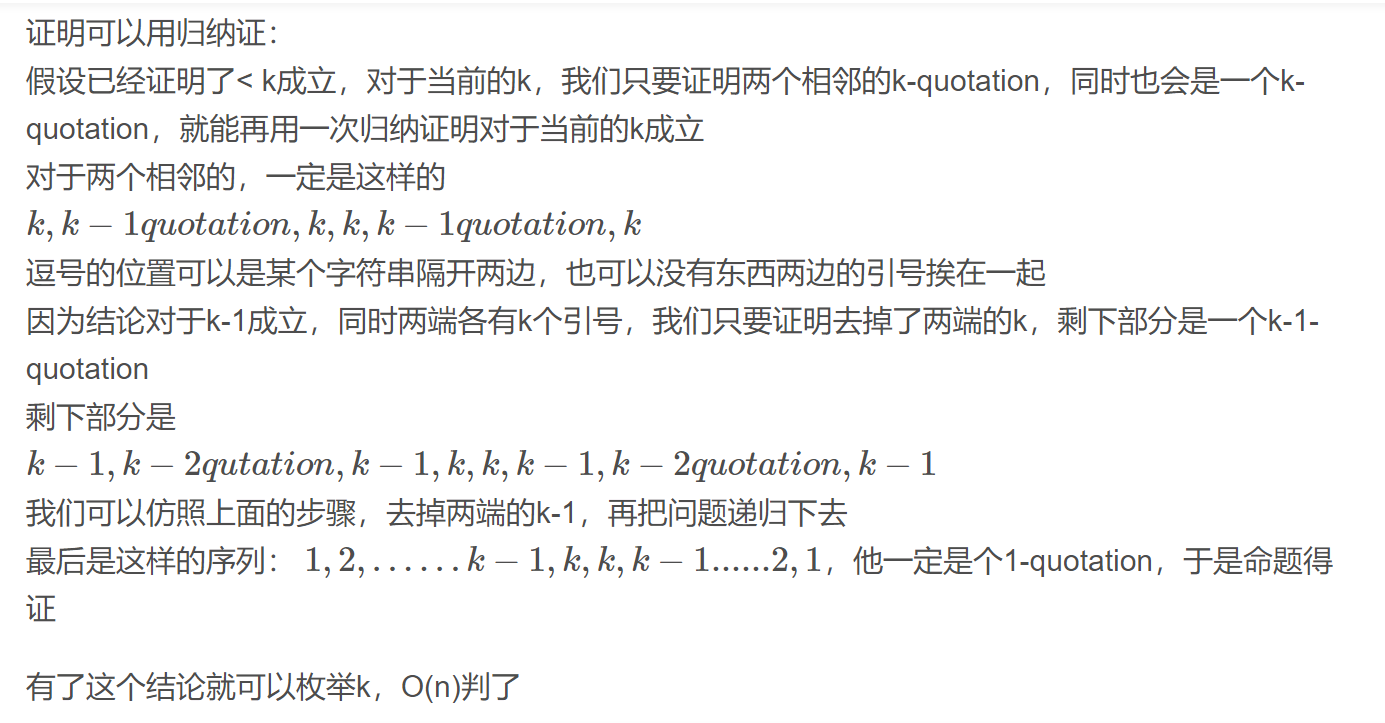# [ICPC2016 WF]String Theory

## 题目描述

1-quotation 指一个以单引号开头并以另一个单引号结尾，而且中间没有单引号的字符串。例如 ‘this is a string

‘ 是一个1-quotation。对于 k＞1，k-quotation 以 k 个单引号开头，以另外 k 个单引号结尾，并且中间嵌套了一个字符串。嵌套在其中的字符串是一系列(k-1)-quotation，它们可能紧接前面，分开，或者跟在若干非引号字符后面。例如”All ‘work’ and no ‘play”’是一个2-quotation。给出一个字符串的描述，请计算它最大可能的嵌套级

（即最大的k使该字符串是一个k-quotation）。

## Solution

= =看错题面了一开始

3,2,1,1,2,2,1,1,2,3

3,2,1,1,(1,1),(1,1),1,1,2,3#include<bits/stdc++.h>
const int N = 105;
int n,b[N],a[N];
int solve(int l,int r,int k){
if(a[l]==0) l++;
if(a[r]==0) r--;
if(k!=1){
if(a[l]>=k&&a[r]>=k){
if(l==r) if(a[l]<2*k) return 0;
a[l]-=k,a[r]-=k;
return solve(l,r,k-1);
}
else return 0;
}
else{
int ans=0;
for(int i=l;i<=r;++i)
ans+=a[i];
return ((ans&1)^1)&&ans!=0;
}
}
int main(){
scanf("%d",&n);
for(int i=1;i<=n;++i)
scanf("%d",&b[i]);
for(int p=std::min(b,b[n]);p>=2;--p){
for(int i=1;i<=n;++i) a[i]=b[i];
if(solve(1,n,p)){
printf("%d",p);
return 0;
}
}
if(n==1&&b==2) puts("1");
else if(n==2&&b==1&&b==1) puts("1");
else puts("no quotation");
return 0;
}